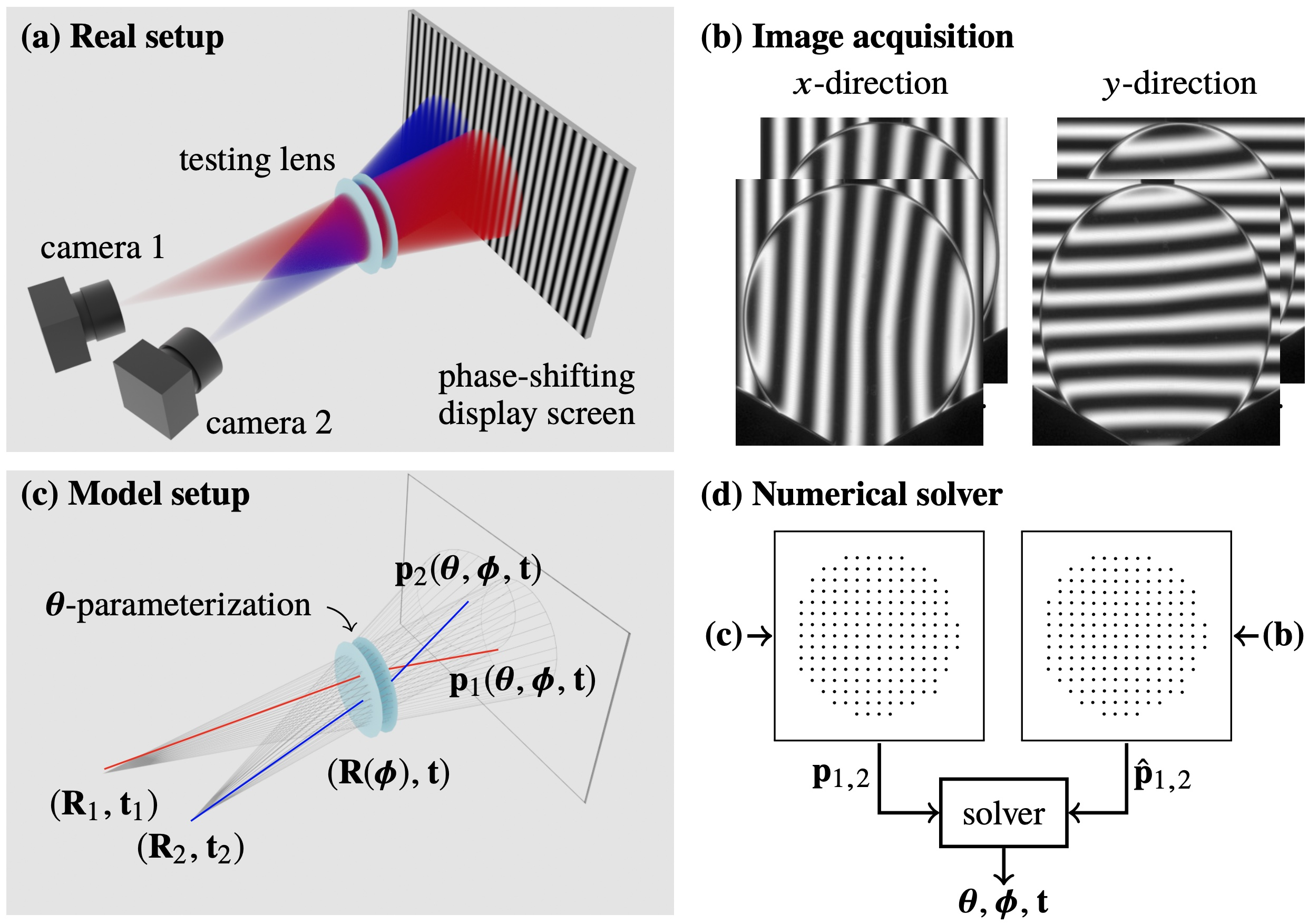## Towards self-calibrated lens metrology by differentiable refractive deflectometry

Congli Wang, Ni Chen, Wolfgang Heidrich
Optics Express 2021Figure: Dual-camera refractive deflectometry for lens metrology. (a) Hardware setup. (b) Captured phase-shifted images, from which on-screen intersections are obtained. (c) A ray tracer models the setup by ray tracing each parameterized refractive surface, obtaining the modeled intersections. (d) Unknown parameters and pose are jointly optimized by minimizing the error between measurement and modeled.

#### Abstract

Deflectometry, as a non-contact, fully optical metrology method, is difficult to apply to refractive elements due to multi-surface entanglement and precise pose alignment. Here, we present a computational self-calibration approach to measure parametric lenses using dual-camera refractive deflectometry, achieved by an accurate, differentiable, and efficient ray tracing framework for modeling the metrology setup, based on which damped least squares is utilized to estimate unknown lens shape and pose parameters. We successfully demonstrate both synthetic and experimental results on singlet lens surface curvature and asphere-freeform metrology in a transmissive setting.

#### Paper

`paper [Wang2021DiffDeflectometry.pdf (2.2MB)]`
`code [GitHub repository]`